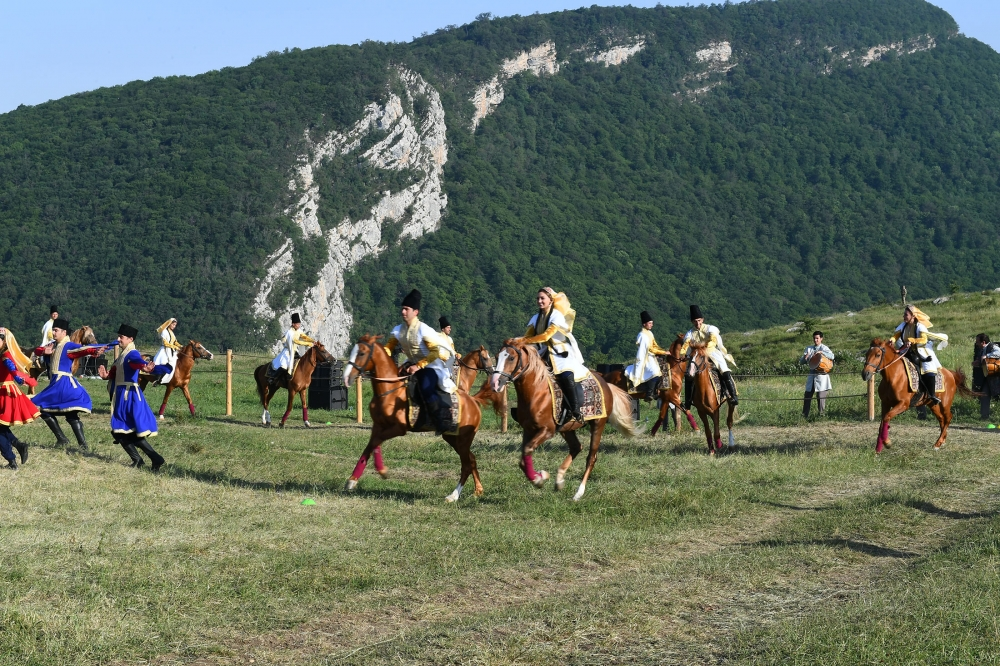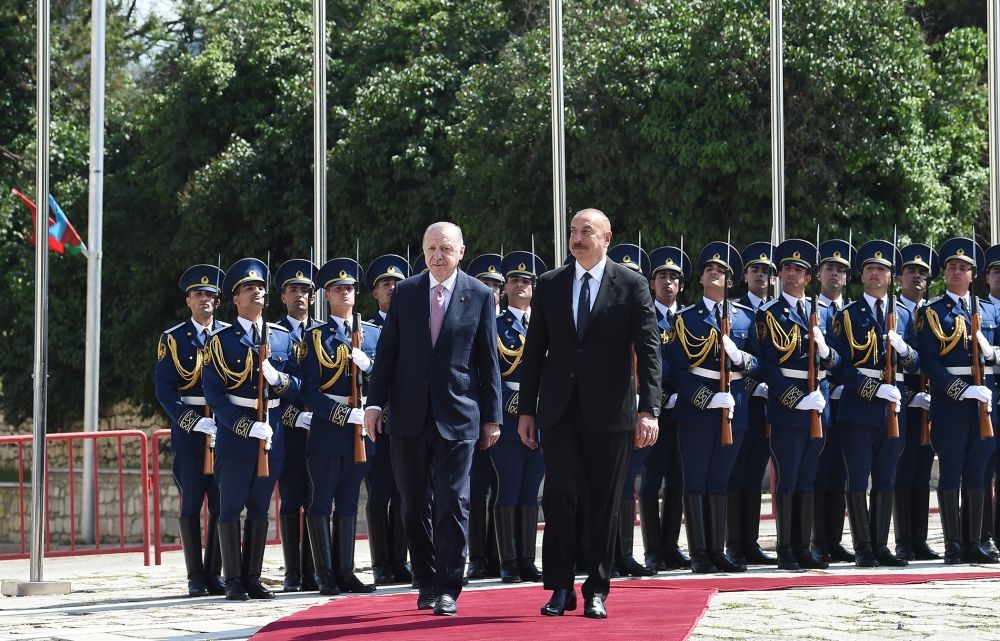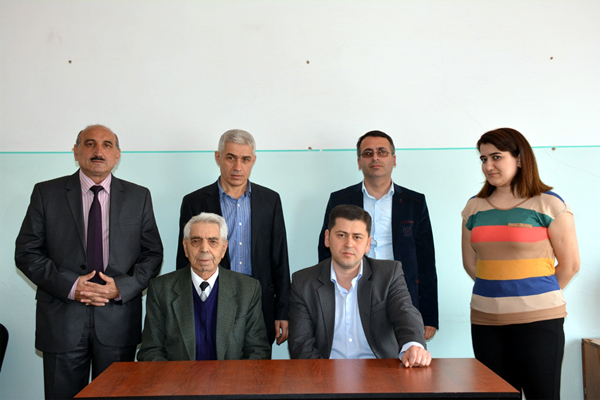Institute of Mathematics and Mechanics

## Karabakh news### “Musical heritage and Karabakh horses on Jidir Duzu plain” composition organized by Heydar Aliyev Foundation was presented in Shusha### Azerbaijan, Turkey signed Shusha Declaration on allied relations## Department of “Theory of functions”Head of the department: Vugar Elman Ismailov Doctor of  Science in mathematics Tel: (+994 12) 5386217 E-mail: vugaris@mail.ru, vugar.ismayilov@imm.az Number of employees: 7 The basic scientific direction of the department: Approximation of multivariate functions by ridge functions, neural networks, linear and nonlinear superpositions, embedding theorems for function spaces. The obtained basic scientific results: 1) Necessary and sufficient conditions for the representation of multivariate functions by sums of functions of fewer variables, linear combinations of ridge functions and linear superpositions were obtained; 2) A Chebyshev type theorem was proved for sums of ridge functions to be extremal to a given continuous function; 3) Explicit formulas for an exact computation of the approximation error and construction of best approximating function were obtained in problems of approximation of multivariate functions by sums of functions of fewer variables and ridge functions in both continuous and integral metrics; 4) It was shown that if each continuous function defined on a compact Hausdorff space is represented by linear superpositions, then all functions on this space possess such representation. In particular, from this result it follows that the famous Kolmogorov superposition theorem and several other superposition theorems, which were proven for continuous functions, are also valid for discontinuous functions. 5) The problem of approximation by neural networks with weights varying on a finite set of directions was considered. Necessary and sufficient conditions for density of such networks were found. 6) A family of new Morrey type spaces was constructed and integral representations for generalized mixed derivatives of functions from these spaces were obtained. By means of these integral representations, differential and differential-difference properties of functions from the constructed spaces were studied. The obtained theoretical results were applied to some higher order differential equations. Main publications on the themes: 1. Vugar E. Ismailov, Approximation by neural networks with weights varying on a finite set of directions, Journal of Mathematical Analysis and Applications, 389 (2012), Issue 1, 72-83. 2.Vugar E. Ismailov, A note on the representation of continuous functions by linear superpositions, Expositiones Mathematicae 30 (2012), Issue 1, 96-101. 3.Vugar E. Ismailov, On the theorem of M Golomb, Proc. Indian Acad. Sci. (Math. Sci.), 119 (2009), no. 1, 45-52. 4.Vugar E. Ismailov, On the representation by linear superpositions, Journal of Approximation Theory 151 (2008), Issue 2 , 113-125. 5.Vugar E. Ismailov, On the approximation by compositions of fixed multivariate functions with univariate functions, Studia Mathematica 183 (2007), 117-126. 6.Vugar E. Ismailov, Representation of multivariate functions by sums of ridge functions, Journal of Mathematical Analysis and Applications 331 (2007), Issue 1, 184-190. 7.Vugar E. Ismailov, Characterization of an extremal sum of ridge functions, Journal of Computational and Applied Mathematics 205 (2007), Issue 1, 105-115. 8. Kadimova Leyla Sh., Najafov Alik M., Theorems on imbedding of functions from the Sobolev-Morrey generalized space, Proc. A. Razmadze Math. Inst. 154 (2010), 97-109. 9. Najafov Alik M., Smooth solutions of a class of quasielliptic equations, Sarajevo J. Math. 3(16) (2007), no. 2, 193-206. 10. Najafov A., Problem on the smoothness of solutions of one class of hypoelliptic equations, Proc. A. Razmadze Math. Inst. 140 (2006), 131-139. 11. Najafov A., Some properties of functions from the intersection of Besov-Morrey type spaces with dominant mixed derivatives, Proc. A. Razmadze Math. Inst. 139 (2005), 71-82. 12. Najafov Alik M., On some properties of the functions from Sobolev-Morrey type spaces, Cent. Eur. J. Math. 3 (2005), no. 3, 496-507. 13. Guliyev V. S., Serbetci, A., Safarov, Z. V., Meda inequality for rearrangements of the B-convolutions and some applications, J. Math. Inequal.  2  (2008), no. 4, 437-447. 14. Guliyev V. S., Safarov Z. V., Serbetci A., On the rearrangement estimates and the boundedness of the generalized fractional integrals associated with the Laplace-Bessel differential operator, Acta Math. Hungar.  119 (2008), no. 3, 201-217. 15. Guliyev V. S., Serbetci A., Safarov Z. V., Inequality of O’Neil-type for convolutions associated with the Laplace-Bessel differential operator and applications, Math. Inequal. Appl. 11 (2008), no. 1, 99-112.
Statistics on COVID-19 in Azerbaijan
• Infected with the virus

517802

• Healed

485232

• Newly infected

0

• Active patient

25655

• The state of death

6915

• Tested

5,117,481